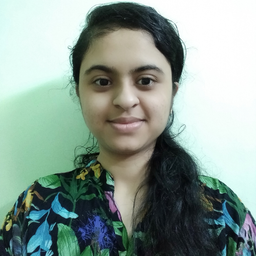Ask your homework questions to teachers and professors, meet other students, and be entered to win $600 or an Xbox Series X 🎉Join our Discord!Numerade Educator ### Problem 82 Hard Difficulty # Find the mean, the median, and the mode of the collection of numbers. $$9,6,10,14,10,3$$ ### Answer ## Mean$=8.67$Median$=9.5$Mode$=10\$

#### Topics

No Related Subtopics

### Discussion

You must be signed in to discuss.

### Video Transcript

in this problem, we have to find me media and word off the given collection off numbers to find the mean we ed the given six numbers and then divide by six. So I mean, is it will do nine. Last six last day Bless 40. Blessed Day Last three divided by six. So this is a point to 50 door divided by six. We just a quad toe 96 divided by three and which gave him Dennis the mixed fraction it to buy three So mean is equal to it. To buy three. Now, to find the media, we like think even numbers in order and find the middle number the numbers can didn't. Then in order. S 36 nine Then then fucking one as these Air six numbers that this even number of numbers we even get to middle numbers. The media numbers are nine and did so to find the media. Bba led nine. Uh huh. And then divide by two. So media is it Will do nine. Last day Divide by two there divided by two. So this is it Will do 90 divided by Do are we Can I take this nine one by do so. Maybe it is equal to 91.2 now to find them. Or we use this order list and find the number that occurs most frequently The number that August most frequently that this two times is there So more is it will do then that this we have found the values off me media on more.Other Schools

#### Topics

No Related Subtopics